# 10kHz to 120MHz VFO / RF Generator with Si5351 and Arduino

VFO/RF generator for use in homebrew radio equipment such as direct conversion and superheterodyne receivers or ham transmitters.

BeginnerWork in progress58,267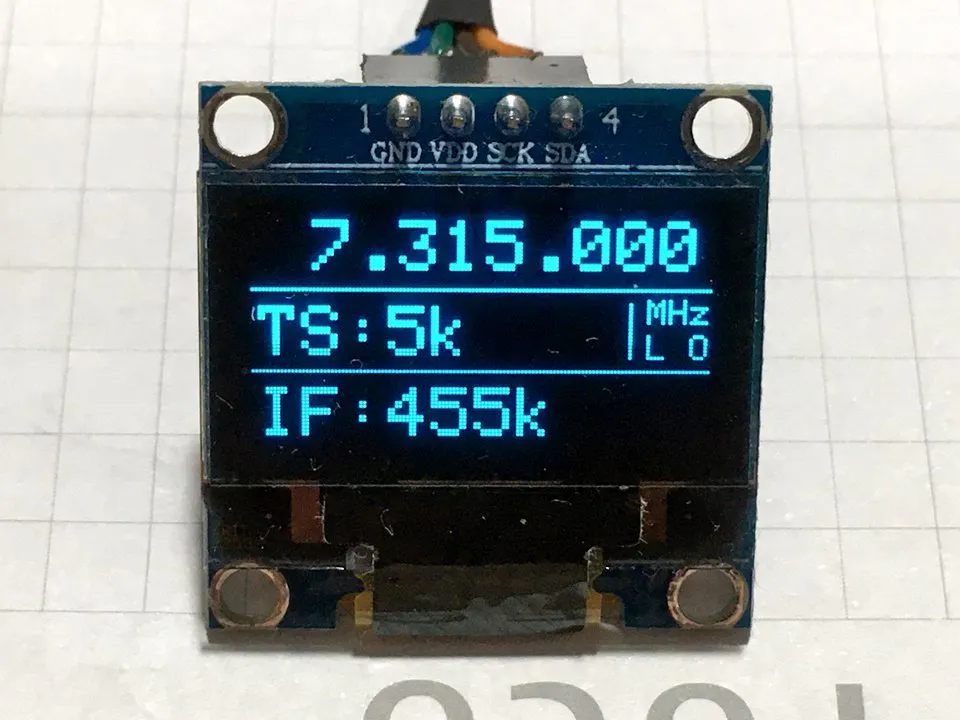## Things used in this project

### Hardware componentsArduino Nano R3
×1
 Adafruit SSD1306 128X64 OLED DISPLAY SSD1306 128X64 OLED DISPLAY
×1
×1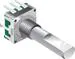Rotary Encoder with Push-Button
×1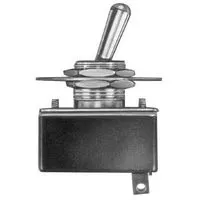Toggle Switch, Toggle
×1
 RCA JACK FOR RF OUTPUT CONECTION
×1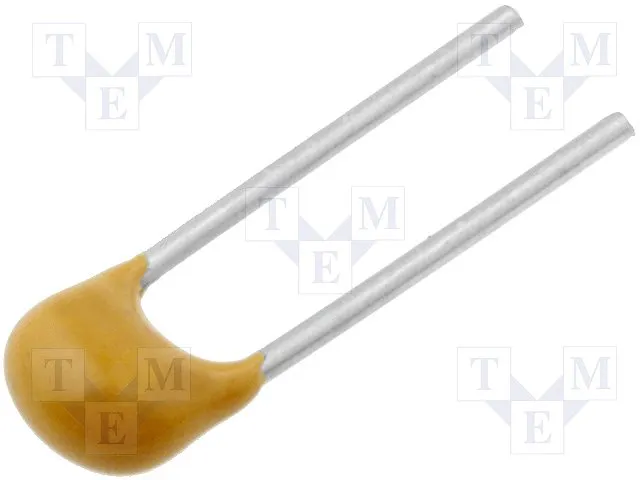Capacitor 100 nF
×2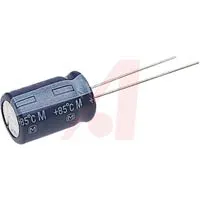Capacitor 10 µF
×1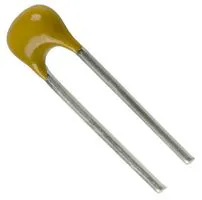Capacitor 10 nF
×2×1

### Software apps and online servicesArduino IDE

## Schematics

### Wiring diagram (schematics)

To interconnect the parts.

## Code

### Sketch SI5351_VFO_RF_GEN_OLED_JCR

C/C++
VFO work. Load it to the Arduino using the IDE.
```/********************************************************************************************************
10kHz to 120MHz VFO / RF Generator with Si5351 and Arduino Nano, with Intermediate Frequency (IF)
offset (+ or -). See the schematics for wiring details. By J. CesarSound - ver 1.0 - Dec/2020.
*********************************************************************************************************/

//Libraries
#include <Wire.h>                 //IDE Standard
#include <Rotary.h>               //Ben Buxton https://github.com/brianlow/Rotary
#include <si5351.h>               //Etherkit https://github.com/etherkit/Si5351Arduino

//User preferences
//------------------------------------------------------------------------------------------------------------
#define IF  0                //Enter your IF frequency, ex: 455 = 455kHz, 10700 = 10.7MHz, 0 = to direct convert receiver or RF generator, + will add and - will subtract IF offfset.
#define FREQ_INIT  7000000   //Enter your initial frequency at startup, ex: 7000000 = 7MHz, 10000000 = 10MHz, 840000 = 840kHz.
#define XT_CAL_F   33000     //Si5351 calibration factor, adjust to get exatcly 10MHz. Increasing this value will decreases the frequency and vice versa.
#define tunestep   A0        //Change the pin used by encoder push button if you want.
//------------------------------------------------------------------------------------------------------------

Rotary r = Rotary(2, 3);
Si5351 si5351;

unsigned long freq = FREQ_INIT;
unsigned long freqold, fstep;
long interfreq = IF;
long cal = XT_CAL_F;
unsigned long long pll_freq = 90000000000ULL;
byte encoder = 1;
byte stp;
unsigned int period = 100;   //millis display active
unsigned long time_now = 0;  //millis display active

ISR(PCINT2_vect) {
char result = r.process();
if (result == DIR_CW) set_frequency(1);
else if (result == DIR_CCW) set_frequency(-1);
}

void set_frequency(short dir) {
if (encoder == 1) {                         //Up/Down frequency
if (dir == 1) freq = freq + fstep;
if (freq >= 120000000) freq = 120000000;
if (dir == -1) freq = freq - fstep;
if (fstep == 1000000 && freq <= 1000000) freq = 1000000;
else if (freq < 10000) freq = 10000;
}
}

void setup() {
Wire.begin();
display.begin(SSD1306_SWITCHCAPVCC, 0x3C);
display.clearDisplay();
display.setTextColor(WHITE);
display.display();

pinMode(2, INPUT_PULLUP);
pinMode(3, INPUT_PULLUP);
pinMode(tunestep, INPUT_PULLUP);

statup_text();

si5351.output_enable(SI5351_CLK0, 1);                  //1 - Enable / 0 - Disable CLK
si5351.output_enable(SI5351_CLK1, 0);
si5351.output_enable(SI5351_CLK2, 0);
si5351.drive_strength(SI5351_CLK0, SI5351_DRIVE_2MA);  //Output current 2MA, 4MA, 6MA or 8MA

PCICR |= (1 << PCIE2);
PCMSK2 |= (1 << PCINT18) | (1 << PCINT19);
sei();

stp = 3;
setstep();
layout();
displayfreq();
}

void loop() {
if (freqold != freq) {
time_now = millis();
tunegen();
freqold = freq;
}

time_now = (millis() + 300);
setstep();
delay(300);
}

if ((time_now + period) > millis()) {
displayfreq();
layout();
}
}

void tunegen() {
si5351.set_freq_manual((freq + (interfreq * 1000ULL)) * 100ULL, pll_freq, SI5351_CLK0);
}

void displayfreq() {
unsigned int m = freq / 1000000;
unsigned int k = (freq % 1000000) / 1000;
unsigned int h = (freq % 1000) / 1;

display.clearDisplay();
display.setTextSize(2);

char buffer = "";
if (m < 1) {
display.setCursor(41, 1); sprintf(buffer, "%003d.%003d", k, h);
}
else if (m < 100) {
display.setCursor(5, 1); sprintf(buffer, "%2d.%003d.%003d", m, k, h);
}
else if (m >= 100) {
unsigned int h = (freq % 1000) / 10;
display.setCursor(5, 1); sprintf(buffer, "%2d.%003d.%02d", m, k, h);
}
display.print(buffer);
}

void setstep() {
switch (stp) {
case 1:
stp = 2;
fstep = 1;
break;
case 2:
stp = 3;
fstep = 10;
break;
case 3:
stp = 4;
fstep = 1000;
break;
case 4:
stp = 5;
fstep = 5000;
break;
case 5:
stp = 6;
fstep = 10000;
break;
case 6:
stp = 1;
fstep = 1000000;
break;
}
}

void layout() {
display.setTextColor(WHITE);
display.drawLine(0, 20, 127, 20, WHITE);
display.drawLine(0, 43, 127, 43, WHITE);
display.drawLine(105, 24, 105, 39, WHITE);
display.setTextSize(2);
display.setCursor(2, 25);
display.print("TS:");
if (stp == 2) display.print("1Hz"); if (stp == 3) display.print("10Hz"); if (stp == 4) display.print("1k");
if (stp == 5) display.print("5k"); if (stp == 6) display.print("10k"); if (stp == 1) display.print("1M");
display.setCursor(2, 48);
display.print("IF:");
display.print(interfreq);
display.print("k");
display.setTextSize(1);
display.setCursor(110, 23);
if (freq < 1000000) display.print("kHz");
if (freq >= 1000000) display.print("MHz");
display.setCursor(110, 33);
if (interfreq == 0) display.print("VFO");
if (interfreq != 0) display.print("L O");
display.display();
}

void statup_text() {
display.setTextSize(1);
display.setCursor(4, 5);
display.print("Si5351");
display.setCursor(4, 20);
display.print("VFO / RF Generator");
display.setCursor(4, 35);
display.print("Version 1.0");
display.setCursor(4, 50);